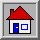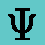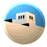Teaching experience

# Instructor - Krzysztof Galicki

## COURSE DESCRIPTION

The main textbook for this course is Algebraic Curves and Riemann Surfaces by Rick Miranda, Graduate Studies in Mathematics, Volume 5, AMS (1995). The lectures will cover the following topics:

## Math 530 - Fall 1998

• Riemann Surfaces: basic definitions

• First few examples: Riemann Sphere, complex tori, graphs of holomorphic functions, and smooth affine plane curves

• Projective curves: smooth projective plane curves and (local) complete intersections

• Functions on Riemann surfaces: holomorphic and meromorphic functions

• Examples of meromorphic functions: Riemann Sphere, complex tori, smooth plane, and smooth projective curves.

• Holomorphic maps between Riemann surfaces: isomorphism and automorphisms, Euler number, Hurwitz's formula

• Further examples examples: lines and conics, hyperelliptic Riemann surfaces, resolving nodes of a plane curve, resolving monomial singularities, cyclic coverings of the line.

• Group actions on Riemann surfaces: quotient map and quotient Riemann surface, ramification of the quotient map, Hurwitz's theorem on automorphisms

• Monodromy: holomorphic maps and coverings via monodromy representations, examples

• Differential forms on Riemann surfaces: holomorphic and meromorphic 1- and 2-forms

• Differential calculus on forms: differentials and wedge products

• Integration on a Riemann surface:residue of a meromorphic 1-form, Stoke's theorem.

## Math 531 - Spring 1999

• Divisors:principal and canonical divisors, degree of a divisor, divisors of holomorphic maps, intersection divisors, and hyperplane divisors

• Linear equivalence of divisors: degree of a smooth projective curve, Bezout's theorem, Pascal's mystic hexagon, Plücker's formula

• The space L(D): complete linear systems and linear systems, pencils and webs

• Divisors and holomorphic maps to projective spaces: defining a holomorphic map via a linear system, criteria for the holomorphic map associated to the complete linear system |D| to be an embedding

• Algebraic curves: function field on an algebraic curve, Laurent tail divisors and Mittag-Leffler problem

• Riemann-Roch theorem and Serre duality: the equality of the three genera

• Applications of Riemann-Roch theorem: genus zero, one, and two curves, Clifford theorem, canonical map and the geometric form of Riemann-Roch, dimension of the moduli space of algebraic curves of genus g, degree of projective curves, general position lemma, Castelnuovo bound, inflection points and Weierstrass points

• Abel's theorem: homology, periods and the Jacobian, the Able-Jacobi map, residue theorem, proof of Abel's theorem, Abel's theorem for genus one curves

## Recommended reading during and after this course

• Complex Algebraic Curves, by Frances Kirwan, Cambridge University Press (1992).

• Basic Algebraic Geometry, Volumes 1&2, by Igor R. Shafarevich, Springer-Verlag (1994).

• Conics and Cubics, A Concrete Introduction to Algebraic Curves, by Robert Bix, Springer-Verlag (1998).

• Algebraic Geometry, A First Course, by Joe Harris, Springer-Verlag (1992).

• Algebraic Geometry, by Robin Hartshorne, Springer-Verlag (1987).

• Principles of Algebraic Geometry, by Philip Griffiths and Joseph Harris, John Wiley (1978).

• Geometry of Algebraic Curves, Volume I, by E. Arbarello, M. Cornalba, P. A. Griffiths, and J. Harris, Springer-Verlag 1985.

• Rational Curves on Algebraic Varieties, by János Kollár, Springer-Verlag (1996).

• Algebraic Geometry. V, A. N. Parishin, and I. R. Shafarevich, Eds., Encyclopedia of Mathematical Sciences, 47, Springer-Verlag (1999).

Return to:My HomepageDepartmen of Mathematics and StatisticsUNM Homepage